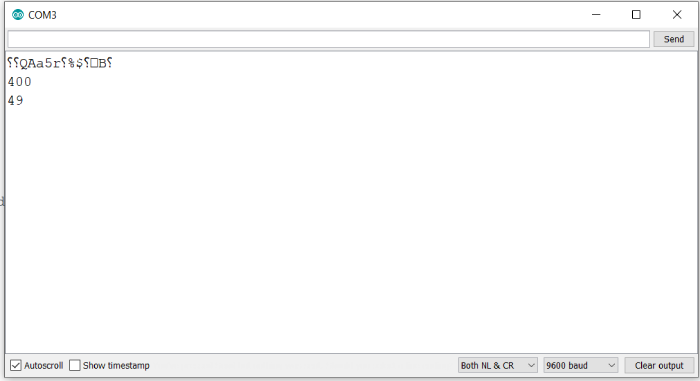# Map a 10-bit number to 8-bit in Arduino

Mappings often have to be performed in Arduino for a variety of reasons. One example would be mapping the 10-bit ADC output to 8-bit to save on storage. A 10-bit number would occupy 2-bytes for storage, whereas an 8-bit number would occupy just one byte and still preserve most of the information of the 10-bit number.

## Syntax

map(value, fromLow, fromHigh, toLow, toHigh)

where, value is the value to be mapped; fromLow and fromHigh are the bounds of the range of the current value; toHigh and toLow are the bounds of the range of the new value.

Thus, if I need to map a 10-bit number to 8-bit number,

## Syntax

map(value, 0, 1023, 0, 255)

This is because a 10-bit number’s minimum value is 0, and maximum is 1023. For an 8-bit number, the min and max values are 0 and 255 respectively.

What you can notice from the syntax is that you can use this function to map a number from any range to any new range. You need not restrict yourself to powers of 2.

## Example

The following example illustrates the use of this function −

void setup() {
// put your setup code here, to run once:
Serial.begin(9600);
Serial.println();

int a = 200;
Serial.println(map(a,0,500,0,1000));
Serial.println(map(a,0,1023,0,255));
}

void loop() {
// put your main code here, to run repeatedly:
}

## Output

The Serial Monitor output is −As you can see, this function can be used for both up-scaling and down-scaling a given number. First, we upscaled the number 200 from the (0,500) range to (0,1000) range. As expected, the function returned 400. In the second case, we down-scaled 200 from (0,1023) range to (0,255) range. The function returned 49, which matches the integer part of 200*255/1023 (=49.85).

You can refer to Arduino’s documentation for more information related to this function: − https://www.arduino.cc/reference/en/language/functions/math/map/